# Data Visualization & Correlation

We implemented the Linear Regression algorithm in the Day4 feature. The data was presented in the table layout but it would be more coherent if it is illustrated in a pictorial format. Python comes with the two most commonly used visualization libraries Matplotlib and Seaborn. Let’s picture how the data is distributed in the Boston housing price dataset.

`import matplotlib.pyplot as pltimport seaborn as sns`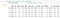Boston housing price dataset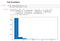Fig 1 — Shows histogram of the column ‘CRIM’

It can be seen that most of the values are condensed around 0 -10 with having a frequency of 450. The height of the histogram reveals where the data is concentrated, the taller the histogram more the concentration.

Next step would be to find the relation between independent and dependent features. Since the algorithm is a linear regression, if there is a strong linear correlation between input and output then we get significant results using this algorithm.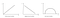Fig 2 — Shows relation between predictors and target

Jointplot in the seaborn library provides a great perception of how input is linked to the output. We can consider the predictor(‘CRIM’) and the target(‘MEDV’).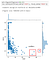Fig 3 — Shows anomalies

There are some anomalies that do not fit into the majority of the data pattern, they are referred to as outliers. In addition to the interconnection, it also shows the individual histograms. Rather than giving each individual column to see the relation, the pair plot provides a way to depict the associations in a single shot for multiple Variables. The diagonal images show individual histograms.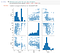Fig4 — shows pair plot for different Variables

Boxplots are the preferred visuals to do a quick check on the existence of the outliers. In the below illustration, we could notice the presence of unusual observations on the right side of the box.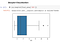Fig 5 — shows box plot with outliers

Box plot uses the Interquartile Range (a statistical approach) to compute the acceptable lower and upper bounds. IQR(interquartile range) = Q3 — Q1 where Q1 is first quantile corresponding to 25% and Q3 is the third quantile corresponding to 75%.

lower bound = Q1 — (1.5 * IQR) & upper bound = Q3 + (1.5 * IQR)

If any training observation falls outside the boundary line, it will be treated as an outlier/anomaly. The presence of too many outliers would deteriorate the model’s efficiency. The reason being, the goal of the model is to learn the patterns in the input data to find the best fit line(y = mx+c) which reduces the overall mean squared error of all the training samples(between the actual and predicted). But if the anomalies are included in the training set, the model tries to learn the pattern from the outlier and thus deviating the best fit line. This produces higher error affecting the predicted result of other properly placed data.

For an ML model to execute well, the data should be normally distributed because the bell-shaped normal curve has most of the data-focused in the central region with meagre extreme points(anomalies).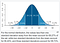Fig 6 — shows Normal distribution taken from wiki

Yet one another approach of discovering the interrelationship between two variables is through Pearson correlation coefficient. The coefficient values range from -1 to 1 following the below interpretation:

1 => Two variables have high positive correlation, -1 => variables are negatively correlated, 0 => there is no correlation between the variables

The intuition behind covariance: In order to understand the correlation, first let’s unravel the concept of covariance. From the formula below and from the name covariance, we can say it is the variance of two variables. If both the variables have variance in the same direction, then the covariance will result in a positive number. On the contrary, if they take exact opposite directions, then the result will be negative.

Note: variance is how each sample differs from the mean.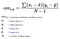Formula for Covariance

Since we are interested only in the direction in which the data vary, we divide the covariance by the respective summation of variance squared of the two variables. This brings the output to the range of -1 to +1.

Heatmap from seaborn is a nice way to explain the correlation between the variables.

`correlation = final_data.corr()plt.figure(figsize=(12,7))sns.heatmap(correlation, annot = True, linewidth = 1)`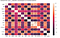Heat map showing the correlation between all the variables

Interpretation of Heatmap: The intensity of the colour represents how well two variables are related to each other. Two types of correlations are observed from the heatmap.

Correlation1: How well the dependent target(‘MEDV’) is linearly correlated to the rest of the independent variables. If there is a strong linkage(1 or -1), then those predictors should be retained as inputs because of the meaningful representation of the output. In our case LSTAT (-0.74), RM(0.7) and PTRATIO(-0.51) are the best predictors when compared to the rest as per linear relation.

Correlation2: Association between the predictors. If two independent variables convey the same information, then one of the variables should be dropped from the input set. To cite an example, when the data is collected by two different teams and some of the input attributes carry similar details.

1. In such cases, retaining only one would save a lot of computation as there is nothing new to learn from the dropped variable.
2. Moreover, in linear regression, we tend to find the coefficients(weights) corresponding to each input variable in relation to the output. If the predictors are correlated to one another(multicollinearity), then change in one input would affect another input and thus losing the independency.

For our case, the variables ‘RAD’ and ‘TAX’ have a correlation coefficient value as 0.91.

The entire code can be found in the GitHub.

Recommended Reading:

https://medium.com/fintechexplained/ever-wondered-why-normal-distribution-is-so-important-110a482abee3

## Nerd For Tech

From Confusion to Clarification

## Nerd For Tech

NFT is an Educational Media House. Our mission is to bring the invaluable knowledge and experiences of experts from all over the world to the novice. To know more about us, visit https://www.nerdfortech.org/. Don’t forget to check out Ask-NFT, a mentorship ecosystem we’ve started

Written by

## Nandhini N

AI Enthusiast | Blogger✍## Nerd For Tech

NFT is an Educational Media House. Our mission is to bring the invaluable knowledge and experiences of experts from all over the world to the novice. To know more about us, visit https://www.nerdfortech.org/. Don’t forget to check out Ask-NFT, a mentorship ecosystem we’ve started

## More From Medium

Medium is an open platform where 170 million readers come to find insightful and dynamic thinking. Here, expert and undiscovered voices alike dive into the heart of any topic and bring new ideas to the surface. Learn more

Follow the writers, publications, and topics that matter to you, and you’ll see them on your homepage and in your inbox. Explore

If you have a story to tell, knowledge to share, or a perspective to offer — welcome home. It’s easy and free to post your thinking on any topic. Write on Medium